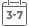# Incompressible Flow and the Finite Element Method, Volume 2, Isothermal Laminar Flow

• Publish Date: 2000-06-09
• Binding: Paperback
• Author: P. M. Gresho;R. L. Sani
• Sale
• \$103.29
• Regular price \$147.97This comprehensive two-volume reference covers the application of the finite element method to incompressible flows in fluid mechanics, addressing the theoretical background and the development of appropriate numerical methods applied to their solution.

Volume One provides extensive coverage of the prototypical fluid mechanics equation: the advection-diffusion equation. For both this equation and the equations of principal interest - the Navier-Stokes equations (covered in detail in Volume Two) - a discussion of both the continuous and discrete equations is presented, as well as explanations of how to properly march the time-dependent equations using smart implicit methods. Boundary and initial conditions, so important in applications, are carefully described and discussed, including well-posedness. The important role played by the pressure, so confusing in the past, is carefully explained.

The book explains and emphasizes consistency in six areas:

* consistent mass matrix

* consistent pressure Poisson equation

* consistent penalty methods

* consistent normal direction

* consistent heat flux

* consistent forces

Fully indexed and referenced, this book is an essential reference tool for all researchers, students and applied scientists in incompressible fluid mechanics.

MORE FROM THIS COLLECTION